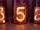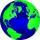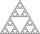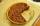# Colors

Willie got birthday 6 colour pens in different colors. How many ways he can give them side by side in pencil?

Result

n =  720

#### Solution:Leave us a comment of example and its solution (i.e. if it is still somewhat unclear...):

Showing 0 comments:Be the first to comment!## Next similar examples:

1. Hexadecimal numberWhat will be hexadecimal number 303 as decimal number?
2. CommitteesHow many different committees of 6 people can be formed from a class of 30 students?
3. SickSick Marcel already taken six tablets, which was a quarter of the total number of pills in the pack. How many pills were in the pack?
4. Big factorialHow many zeros end number 116! ?
5. Permutations without repetitionFrom how many elements we can create 720 permutations without repetition?
6. DisjointHow many elements have union and intersection of two disjoint sets when the first have 1 and secodn 8 elements.
7. Theorem proveWe want to prove the sentense: If the natural number n is divisible by six, then n is divisible by three. From what assumption we started?
8. MidpointsTriangle whose sides are midpoints of sides of triangle ABC has a perimeter 45. How long is perimeter of triangle ABC?
9. WithdrawalIf I withdrew 2/5 of my total savings and spent 7/10 of that amount. What fraction do I have in left in my savings?
10. Cupcakes 2Susi has 25 cupcakes. She gives 4/5. How much does she have left?
11. Unknown number 11That number increased by three equals three times itself?
12. RoundingThe following numbers round to the thousandth:
13. Round it0.728 round to units, tenths, hundredths.
14. TeacherTeacher Rem bought 360 pieces of cupcakes for the outreach program of their school. 5/9 of the cupcakes were chocolate flavor and 1/4 wete pandan flavor and the rest were vanilla flavor. How much more chocolate flavor cupcakes than vanilla flavor?
15. Find xSolve: if 2(x-1)=14, then x= (solve an equation with one unknown)
16. Pizza 4Marcus ate half pizza on monday night. He than ate one third of the remaining pizza on Tuesday. Which of the following expressions show how much pizza marcus ate in total?
17. Pie IIVili ate three pieces of pie. If it pieces is 1/8 how much pie did he eat?